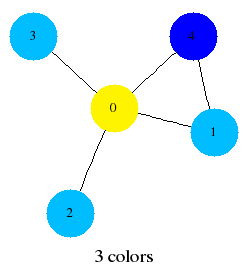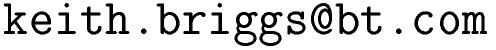Keith Briggshome · papers · thesis · talks · meetings · records · maths notes · software « · languages · music · travel · cv · students · maps · place-names · people · photos · links · ex libris · site map### The very_nauty graph library - version 1.1

This is a C library of graph algorithms, especially targeted at very fast generation of random graphs, and exact clique number and chromatic number computation. The name comes from the fact that it is designed to be compatible with Brendan McKay's nauty software, which is mainly concerned with graph generation and isomorphism testing. Also, since the problems here are NP-complete, it's a bit naughty to even attempt them. But the basic philosophy is that such calculations become more and more feasible as computers get faster. This gives us powerful ways of checking conjectures in graph theory, and also for checking the accuracy of asymptotic formulas. In practice, it's possible to use the exact algorithms on graphs with up to a few hundred nodes. For some results computed with this software see:

#### basic graph functions and macros

All graphs are simple and undirected. They are represented internally by dynamically allocated adjacency lists.

 graph_t type of a graph void set_adj_list_initial_size(size_t sz) set initial size of adjacency lists (defaults to 8) graph_t graph_new(unsigned int n); return a new empty graph with n nodes labelled 0..n-1 void graph_clear(graph_t g); destroy a graph void graph_empty(graph_t g); set to the empty graph void graph_add_edge(graph_t g,node_t i,node_t j); add undirected edge i-j to the graph void graph_del_edge(graph_t g,node_t i,node_t j); delete edge i-j from the graph int graph_has_edge(graph_t g,node_t i,node_t j); 1 if edge i-j is present in g, else 0 void graph_add_node(graph_t g); add a node to the graph nnodes(g) number of nodes in g nedges(g) number of edges in g graph_node_degree(graph_t g, node_t i); degree of a node int graph_min_degree(graph_t g); minimum degree of a node in g int graph_max_degree(graph_t g); maximum degree of a node in g double graph_mean_degree(graph_t g); return mean degree of the nodes of g void graph_show(graph_t g); list edges of g to stdout void graph_make_dotfile(graph_t g, char* fn); write a dotfile suitable for processing by graphviz void graph_make_dotfile_colored(graph_t g, char* fn); write a colored dotfile suitable for processing by graphviz void graph_to_dimacs(graph_t g, char* fn); write graph to a file named fn in DIMACS format void graph_to_theta(graph_t g, char* fn); write graph to a file named fn in a format suitable for reading by Benson's Lovász theta program int graph_nclusters(graph_t g); the number of clusters (connected components) in g int graph_connected(graph_t g); 1 if g is connected, else 0 cluster(g,i) cluster to which node i belongs (valid only after a call to graph_greedy_color or graph_nclusters) int* graph_cluster_sizes(graph_t g) return a (0-terminated) list of cluster sizes, largest first int graph_max_cluster(graph_t g) size of largest cluster (graph is connected if this equals nnodes(g))

#### random graph generation

For more than a few graphs, it's better to use the iterators in the next section (especially for GRG).

 void graph_gnp(graph_t g, double p); generate an instance of the random graph G{n,p} void graph_gnm(graph_t g, int m); generate an instance of the random graph G(n,m) void graph_grg(graph_t g, double r); generate an instance of the geometric random graph GRG(n,r). This has n nodes in the unit square, with each pair linked if their separation is less than r void graph_lognormal_grg(graph_t g, double r, double alpha); nodes a distance d apart are joined with probability (1-erf(log(d/r)/alpha))/2 void graph_grg_torus(graph_t g, double r); as graph_grg, but in a unit-area torus instead of a square void graph_lognormal_grg_torus(graph_t g, double r, double alpha); nodes a torus distance d apart are joined with probability (1-erf(log(d/r)/alpha))/2

#### random graph iterators

When using these, an indefinitely large sample is generated from the specified ensemble, and f is called for each graph. f should return 0 to terminate the iteration. Arbitrary client data or parameters may be passed to f through cd.

 graph_gnp_iterator(unsigned int n, double p, int f(graph_t,int,void*), void* cd); G{n,p} graph_gnm_iterator(unsigned int n, unsigned long m, int f(graph_t,int,void*), void* cd); G(n,m) int graph_grg_torus_iterator(unsigned int n, double r, int f(graph_t,int,void*), void* cd); GRG(n,r) int graph_random_line_graph_iterator(unsigned int nlines, int f(graph_t,int,void*), void* cd) model: place nlines dots uniformly in the unit square; put a line of random slope through each dot; intersections of these lines are the nodes of the graph; line segments between intersections are the edges of the graph int graph_random_line_poisson_graph_iterator(double tau, int f(graph_t,int,void*), void* cd) as above, but a Poisson number (mean=4*tau/pi) of nodes in the square

#### clique number (ω) computation

 int graph_clique_number(graph_t g); compute the exact clique number of g. Warning: this can take a long time for large graphs

#### complementation and switching

 void graph_complement(graph_t gc, graph_t g); complement g void graph_local_complement(graph_t g, node_t i); in-place local complementation at node i; i.e. subgraph N_i(g) is complemented, where N_i(g) is the set of neighbours of i void graph_switching(graph_t g, node_t i); in-place switching at node i; i.e. flip dyads incident on i

#### coloring and chromatic number (χ) computation

 int graph_greedy_color(graph_t g,int perm[]); color the nodes of g in the node order given by perm, which must be a permutation of 0..nnodes(g)-1, or NULL, in which case the identity permutation is used. This returns a rapidly computable upper bound to the chromatic number int graph_sequential_color(graph_t g,int perm[],int ub); color the nodes of g in node order given by perm, aborting as soon as more than ub colors are used (in which case -1 is returned). This returns an upper bound to the chromatic number int graph_sequential_color_repeat(graph_t g, int n); color g's nodes n times in different random orders, and return the number of colors used in the best coloring found. The larger n is, the tighter the upper bound to the chromatic number is likely to be int graph_chromatic_number(graph_t g,clock_t timeout); compute the exact vertex chromatic number of g. If the timeout value (in seconds) is not zero and is reached, return -1. Warning: this can take a long time for large graphs int graph_edge_chromatic_number(graph_t g,clock_t timeout); compute the exact edge chromatic number of g. If the timeout value (in seconds) is not zero and is reached, return -1. Warning: this can take a long time for large graphs color(g,i) color of node i in g (valid only after calling one of the coloring functions) int graph_ncolors(graph_t g); number of colors used in a coloring of g int graph_check_coloring(graph_t g); check that a node coloring is valid

#### histogram functions

Some convenience functions for collecting statistics (data restricted to non-negative integer values)...

 histogram_t histogram_new(char* lbl); make a new histogram labelled lbl void histogram_empty(histogram_t); reset a histogram to all zeros void histogram_add(histogram_t h, int i); add datum i to h void histogram_clear(histogram_t h); destroy h void histogram_show(histogram_t h); print h to stdout void histogram_write(FILE*,histogram_t h); write h to file double histogram_mean(histogram_t h); compute the mean of h int histogram_max(histogram_t h); maximum value in h double histogram_variance(histogram_t h); compute the variance of h unsigned int histogram_mode(histogram_t h); compute the mode of h int histogram_biggest_done(histogram_t h, double eps); return 1 if the modal value has relative standard error less than eps, else 0 int histogram_mean_done(histogram_t h, double eps); return 1 if the mean has relative standard error less than eps, else 0 double histogram_quantile(histogram_t h, double q); compute the qth quantile of h (q=0.5 for the median) histogram_t graph_geng_reader(FILE* f, int op(graph_t), char* lbl); iterate over graphs in file f (in graph6 or sparse6 format), applying op to each and returning a histogram histogram_t graph_showg_reader(FILE* f, int op(graph_t), char* lbl); iterate over graphs in file f (in "showg -l0 -e" format), applying op to each and returning a histogram

• get very_nauty-1.1.tgz
• tar zxf very_nauty-1.1.tgz
• cd very_nauty-1.1
• make
• sudo make install

#### simple library usage example

• cat example_03.c
```#include "vn_graph.h"

int main() {
graph_t g=graph_new(5);
graph_show(g);
printf("chi=%d\n",graph_chromatic_number(g,0));
graph_make_dotfile_colored(g,"example_03.dot");
printf("number of clusters=%d\n",graph_nclusters(g));
printf("nnodes=%d number of clusters=%d\n",nnodes(g),graph_nclusters(g));
graph_clear(g);
return 0;
}
```
• gcc -I/usr/local/include example_03.c -o example_03 -L/usr/local/lib -lvn_graph -lm
• ./example_03
```0: 1 2 3 4
1: 0 4
2: 0
3: 0
4: 0 1
chi=3
example_03.dot written: now do "neato -Tps example_03.dot | gv -"
number of clusters=1
nnodes=6 number of clusters=2
```
• neato -Tpng example_03.dot > example_03.png#### stand-alone programs

The programs vn_graph_omega and vn_graph_chi are provided for computing clique and (vertex) chromatic number of geng output. Also vn_graph_chi_dash computes the edge chromatic number.

Examples:

• Compute the clique number of all 8-node graphs:
```kbriggs:~/very_nauty-1.1> geng 8 | time ./vn_graph_omega
>A geng -d0D7 n=8 e=0-28
>Z 12346 graphs generated in 0.03 sec
graph_omega 12346 items; mode=3 average=3.528997 variance=0.4996 stdev=0.7069 stderr=0.006362
1          1   0.000081    0.008% se=nan
2        409   0.033128    3.313% se=0.0104
3       6021   0.487688   48.769% se=0.000793
4       4985   0.403775   40.377% se=0.0012
5        842   0.068200    6.820% se=0.000608
6         80   0.006480    0.648% se=0.000205
7          7   0.000567    0.057% se=6.62e-05
8          1   0.000081    0.008% se=nan
0.15user 0.02system 0:00.25elapsed 67%CPU
```
• Estimate the distribution of chromatic number in G(50,1/5):
```kbriggs:~/very_nauty-1.1> genrang -P20/100 50 10000 | time ./vn_graph_chi
graph_chi 10000 items; mode=5 average=5.010700 variance=0.01239 stdev=0.1113 stderr=0.001113
4          9   0.000900    0.090% se=0.000298
5       9875   0.987500   98.750% se=1.26e-05
6        116   0.011600    1.160% se=0.000198
11.56user 0.33system 0:13.53elapsed 87%CPU
```
• Compute the chromatic number of all 10-node connected graphs regular of degree 4:
```kbriggs:~/very_nauty-1.1> geng -c -d4 -D4 10 | ./vn_graph_chi
>A geng -cd4D4 n=10 e=20
>Z 59 graphs generated in 0.01 sec
graph_chi 59 items; mode=4 average=3.491525 variance=0.2887 stdev=0.5373 stderr=0.06995
2          1   0.016949    1.695% se=nan
3         28   0.474576   47.458% se=0.0207
4         30   0.508475   50.847% se=0.0246
```
• Compute the edge chromatic number of all 10-node connected triangle-free graphs:
```kbriggs@europa:~/very_nauty-1.1> geng -c -t 10 | time ./vn_graph_chi_dash
>A geng -ctd1D9 n=10 e=9-25
>Z 9832 graphs generated in 0.04 sec
graph_chi_dash 9832 items; mode=4 mean=4.440602 var=0.5836 stdev=0.7639 stderr=0.007704
2          2   0.000203    0.020% se=4.48e-05
3        694   0.070586    7.059% se=0.000612
4       4953   0.503763   50.376% se=0.00121
5       3435   0.349369   34.937% se=0.000571
6        659   0.067026    6.703% se=0.00225
7         80   0.008137    0.814% se=0.00719
8          8   0.000814    0.081% se=0.0323
9          1   0.000102    0.010% se=nan
0.14user 0.00system 0:00.26elapsed 55%CPU
```

#### acknowledgements

• Brendan McKay for nauty, from which I borrowed code to read graph6 and sparse6 format.
• Kevin O'Neill for the original version of the exact clique number algorithm. I modified this to use dynamic memory allocation and made various other improvements.
• Michael Trick for the original version of the exact chromatic number algorithm. I modified this to use dynamic memory allocation and made various other improvements.
• Linlin Song for beta-testing
This website uses no cookies. This page was last modified 2013-05-12 10:17 by.